# JP2011258178A - Numerical control device for multispindle processing machine - Google Patents

## Info

Publication number
JP2011258178A
JP2011258178A JP2011016952A JP2011016952A JP2011258178A JP 2011258178 A JP2011258178 A JP 2011258178A JP 2011016952 A JP2011016952 A JP 2011016952A JP 2011016952 A JP2011016952 A JP 2011016952A JP 2011258178 A JP2011258178 A JP 2011258178A
Authority
JP
Japan
Prior art keywords
axis
tool
rotation
axes
interpolation
Prior art date
Legal status (The legal status is an assumption and is not a legal conclusion. Google has not performed a legal analysis and makes no representation as to the accuracy of the status listed.)
Granted
Application number
JP2011016952A
Other languages
Japanese (ja)
Other versions
JP4847613B2 (en
Inventor
Osamu Hanaoka
Soichiro Ide
Toshiaki Otsuki
Takafumi Sasaki

Original Assignee
Fanuc Ltd
ファナック株式会社
Priority date (The priority date is an assumption and is not a legal conclusion. Google has not performed a legal analysis and makes no representation as to the accuracy of the date listed.)
Filing date
Publication date
Priority to JP2010108639 priority Critical
Priority to JP2010108639 priority
Application filed by Fanuc Ltd, ファナック株式会社 filed Critical Fanuc Ltd
Priority to JP2011016952A priority patent/JP4847613B2/en
Publication of JP2011258178A publication Critical patent/JP2011258178A/en
Application granted granted Critical
Publication of JP4847613B2 publication Critical patent/JP4847613B2/en
Active legal-status Critical Current
Anticipated expiration legal-status Critical

• 238000004364 calculation method Methods 0.000 claims description 60
• 238000003754 machining Methods 0.000 claims description 33
• 238000011156 evaluation Methods 0.000 claims description 22
• 238000000034 method Methods 0.000 claims description 5
• 230000001276 controlling effect Effects 0.000 abstract description 3
• 238000010586 diagram Methods 0.000 description 8
• 238000006243 chemical reaction Methods 0.000 description 5
• 238000004458 analytical method Methods 0.000 description 2
• 239000011159 matrix material Substances 0.000 description 2
• 229940057373 C-Time Drugs 0.000 description 1
• 230000001133 acceleration Effects 0.000 description 1
• 230000000875 corresponding Effects 0.000 description 1
• 238000005516 engineering process Methods 0.000 description 1
• 230000000737 periodic Effects 0.000 description 1
• 238000004088 simulation Methods 0.000 description 1
• 230000017105 transposition Effects 0.000 description 1

## Images

•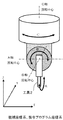•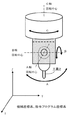•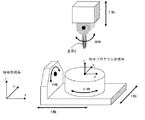•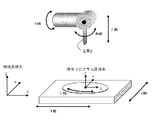•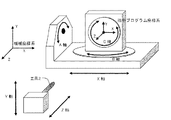•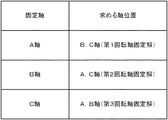•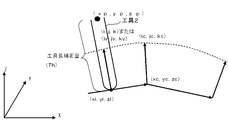•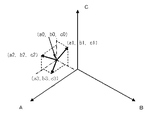•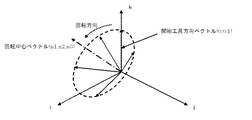•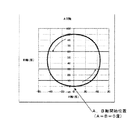•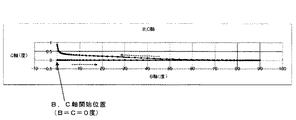•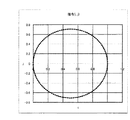•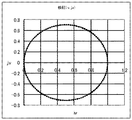•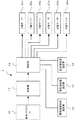•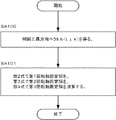•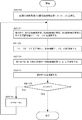## Classifications

• GPHYSICS
• G05CONTROLLING; REGULATING
• G05BCONTROL OR REGULATING SYSTEMS IN GENERAL; FUNCTIONAL ELEMENTS OF SUCH SYSTEMS; MONITORING OR TESTING ARRANGEMENTS FOR SUCH SYSTEMS OR ELEMENTS
• G05B19/00Programme-control systems
• G05B19/02Programme-control systems electric
• G05B19/18Numerical control [NC], i.e. automatically operating machines, in particular machine tools, e.g. in a manufacturing environment, so as to execute positioning, movement or co-ordinated operations by means of programme data in numerical form
• G05B19/41Numerical control [NC], i.e. automatically operating machines, in particular machine tools, e.g. in a manufacturing environment, so as to execute positioning, movement or co-ordinated operations by means of programme data in numerical form characterised by interpolation, e.g. the computation of intermediate points between programmed end points to define the path to be followed and the rate of travel along that path
• G05B19/4103Digital interpolation
• GPHYSICS
• G05CONTROLLING; REGULATING
• G05BCONTROL OR REGULATING SYSTEMS IN GENERAL; FUNCTIONAL ELEMENTS OF SUCH SYSTEMS; MONITORING OR TESTING ARRANGEMENTS FOR SUCH SYSTEMS OR ELEMENTS
• G05B2219/00Program-control systems
• G05B2219/30Nc systems
• G05B2219/49Nc machine tool, till multiple
• G05B2219/49286Two rotations gives cartesian coordinates, compact construction

## Abstract

PROBLEM TO BE SOLVED: To provide a numerical control device for controlling tool tip point on a multispindle processing machine with three spindles.SOLUTION: A numerical control unit 1 performing processing by commanding tool tip-point position and tool orientation on a command program coordinate system comprises: a spindle multiple solution operation section 15 that interpolates the tool orientation command for every interpolation cycle to acquire interpolation tool orientation vectors, and operates to acquire multiple solutions for three spindles from the interpolation tool orientation vectors; a spindle position operation section 17 that synthesizes the multiple solutions to arithmetically acquires the positions of three spindles; and a linear spindle position operation section 19 that interpolates the tool tip-point positions which are commanded for every interpolation cycle to acquire interpolation tool tip-point positions, integrates tool length correction amount to verification tool orientation vectors based on the interpolation tool orientation vectors or three spindle positions acquired by the spindle position operation section, and adds the same to the interpolation tool tip-point positions to operate three linear spindle positions. The machine shifts to the spindle positions acquired by the spindle position operation section 17 and to the linear spindle positions acquired by the linear spindle position operation section 19.

## Description

The present invention relates to a numerical control device that controls a machine tool, and in particular, machining by a mechanism that rotates a table or a tool head with at least three linear axes and three rotation axes with respect to a workpiece (workpiece) attached to a table. The present invention relates to a numerical control device that controls a multi-axis machining machine.
In addition to three linear axes (X, Y, Z axes), five-axis processing machines having two rotation axes have become widespread. The 5-axis processing machine includes a head rotation type having two rotation axes on the tool head side, a table rotation type having two rotation axes on the table side, one rotation axis on the tool head side, and a rotation axis 1 on the table side. There are mixed types with axes. Then, on the command program coordinate system, the tool tip point is commanded by the linear axis position, the tool direction command is used to command the relative tool direction with respect to the workpiece (workpiece), and the workpiece tip point is controlled. A control technology for control is known (see Patent Document 1).
However, in the 5-axis machine, there is a singular point where the rotation axis operates greatly. Therefore, for example, Patent Document 2 discloses a method for determining whether or not a singular point is approached, and generating a virtual axis when the singular point is approached to avoid a large movement of the rotating shaft near the singular point. Yes.
The method disclosed in Patent Document 2 is considered to have the following problems.
First, even if a virtual axis is generated and the amount of movement is obtained, the virtual axis does not actually operate because it is not an actually existing axis. Therefore, even if a large operation of the rotating shaft can be avoided, it is considered that an error occurs at the machining point.
Secondly, in order to determine whether or not a singular point has been approached, there may be cases where one is determined to be close to a singular point and the other is not determined to be close to a singular point even though the routes are almost the same. In this case, the operation of the tool is greatly different in spite of almost the same machining path.
As a result, the processed surface may be streaked or have a step.
Japanese Patent Laid-Open No. 2003-195917 Special table 2008-546099 gazette
Recently, a tool head for a rotary head type multi-axis machine having a three-axis rotation axis as shown in FIGS. 1 and 2 has been used. Further, a mixed multi-axis machine (see FIG. 3) having two table rotation axes and one head rotation axis is also used. Further, a mixed multi-axis machining machine (see FIG. 4) having a table rotation 1 axis and a head rotation 2 axes and a table rotation multi-axis machining machine (see FIG. 5) having a table rotation 3 axes are also considered as the machine configuration. It is done. However, there has been no technical idea of performing tool tip point control by numerical control for a multi-axis machine tool as described above.
Accordingly, an object of the present invention is to provide a tool tip according to a linear axis position on a command program coordinate system as a numerical control device that performs tool tip point control in a multi-axis machining machine having three rotation axes as described above. In the tool tip point control that controls the machining by commanding a point position and commanding a relative tool direction command with respect to the workpiece (workpiece), it is difficult to avoid it with a 5-axis machine with two rotation axes This is to avoid the large movement of the rotating shaft near the singular point without causing a machining error. Even in almost the same machining path, one is judged to be near the singular point and the other is To provide a numerical control device capable of preventing a large difference in operation in a case where it is not determined to be close to a singular point even though the machining paths are almost the same. In other words, the present invention is to provide a numerical control apparatus capable of making the path that avoids a large motion of the rotating shaft near the singular point almost the same if the machining paths are almost the same.
The present invention provides a numerical control device having the following characteristics as a numerical control device that performs tool tip point control in order to realize the above-mentioned problem in a multi-axis machining machine having three rotation axes as described above. To do.
The invention according to claim 1 of the present application is directed to a command program coordinate system in a multi-axis processing machine that processes a workpiece mounted on a table by a mechanism that rotates a table or a tool head with at least three linear axes and three rotation axes. In a numerical controller that performs machining by commanding a tool tip point position and commanding a tool direction command on the system, an interpolation tool direction vector is obtained by interpolating the tool direction command at each interpolation cycle, A rotation axis multiple solution calculation unit that calculates a plurality of solutions of the three rotation axes from an interpolation tool direction vector; a rotation axis position calculation unit that calculates the rotation axis position of the three rotation axes by combining the plurality of solutions; The tool tip point position commanded at each interpolation cycle is interpolated to obtain the interpolation tool tip point position, the interpolation tool tip point position, the tool length correction amount, and the three rotation axes of the rotation axis A linear axis position calculation unit that calculates a linear axis position of the three linear axes on the machine coordinate system from the position, and moves the three rotation axes to the rotation axis position obtained by the rotation axis position calculation unit. The numerical control device is characterized in that the three linear axes move to the linear axis position obtained by the linear axis position calculation unit.
In the invention according to claim 2, the linear axis position calculation unit adds the tool length correction amount to the interpolation tool direction vector, adds the tool length correction amount to the interpolation tool tip point position, and adds the three linear axes on the machine coordinate system. The numerical control device according to claim 1, wherein the numerical control device is a linear axis position calculation unit that calculates a linear axis position.
According to a third aspect of the present invention, the rotation axis position calculation unit calculates the rotation axis position of the three rotation shafts by combining the plurality of solutions and obtains the calculated rotation axis position of the three rotation axes. A rotation axis position calculation unit for obtaining a verification tool direction vector, which is a tool direction vector to be obtained, and the linear axis position calculation unit adds the tool length correction amount to the verification tool direction vector and adds it to the interpolation tool tip point position The numerical controller according to claim 1, wherein the numerical controller is a linear axis position calculation unit that calculates linear axis positions of the three linear axes on the machine coordinate system.
According to a fourth aspect of the present invention, the linear axis position calculation unit is configured to calculate a machine coordinate system from the tool length correction amount, the rotation axis position of the three rotation axes, and the interpolation tool tip point position according to Formula 10-3 described later. The numerical control device according to claim 1, wherein the numerical control device is a linear axis position calculation unit that calculates linear axis positions of the three linear axes above.
In the invention according to claim 5, the plurality of solutions are a first rotating shaft fixed solution when the first rotating shaft is not operated, a second rotating shaft fixed solution when the second rotating shaft is not operated, 5. The numerical controller according to claim 1, wherein the numerical control device is a third rotating shaft fixed solution when the three rotating shafts do not operate.
In the invention according to claim 6, the plurality of solutions are the first rotation axis fixed solution and the second rotation when the first rotation axis is moved by the movement amount of the first rotation axis obtained in the interpolation cycle one cycle before. When the axis moves by the amount of movement of the second rotation axis obtained in the previous interpolation cycle, the third rotation axis is fixed, and the third rotation axis is the third rotation axis obtained in the previous interpolation cycle. 5. The numerical control device according to claim 1, wherein the numerical control device is a third rotating shaft fixed solution when moving by a moving amount.
In the invention according to claim 7, the rotation axis position calculation unit calculates an evaluation value for evaluating that the movement amount is small with respect to the plurality of solutions, and adds the evaluation value to the movement amount by the plurality of solutions. The numerical control device according to claim 1, wherein the numerical control device is a rotation axis position calculation unit that combines the two.
According to an eighth aspect of the present invention, the rotation axis position calculation unit obtains the verification tool direction vector from the calculated rotation axis positions of the three rotation axes, and whether the difference from the interpolation tool direction vector is within a set tolerance. The rotation axis position calculation unit repeats the calculation of the rotation axis position for recalculation so as to evaluate whether the movement amount is small for the evaluation value if it is not within the tolerance. It is a numerical control apparatus as described in any one of 1-7.
The invention according to claim 9 is the multi-axis processing machine according to any one of claims 1 to 8, wherein the multi-axis processing machine is a multi-axis processing machine that rotates a tool head with the three rotation axes. It is a numerical control device.
The invention according to claim 10 is that the multi-axis machine is a multi-axis machine that rotates the table with two rotation axes among the three rotation axes and rotates the tool head with another rotation axis. The numerical control apparatus according to claim 1, wherein:
According to an eleventh aspect of the present invention, the multi-axis processing machine is a multi-axis processing machine that rotates a tool head with two rotation axes among the three rotation axes and rotates a table with one rotation axis. The numerical control apparatus according to claim 1, wherein:
The invention according to claim 12 is the numerical value according to any one of claims 1 to 8, wherein the multi-axis machine is a multi-axis machine that rotates a table with the three rotation axes. It is a control device.
The invention according to claim 13 is the numerical control apparatus according to any one of claims 1 to 12, wherein the tool direction command is commanded by a tool direction vector.
The invention according to claim 14 is the numerical control device according to any one of claims 1 to 12, wherein the tool direction command is commanded by a position of two rotary shafts.
The invention according to claim 15 is the numerical control device according to any one of claims 1 to 12, wherein the tool direction command is commanded by a position of three rotation shafts.
According to the present invention, it is possible to provide a numerical control device that performs tool tip point control in a multi-axis machine with three rotation axes.
In particular, in the tool tip point control in which the tool tip point position is commanded by the linear axis position on the command program coordinate system and the tool is commanded relative to the workpiece (workpiece) to control the machining, the rotary shaft 2 The large movement of the rotating shaft near the singular point, which was difficult to avoid with a 5-axis machine equipped with a shaft, can be avoided without generating a machining error. In the case where one is determined to be close to a singular point and the other is not determined to be close to a singular point, it is possible to provide a numerical control device that can prevent a large difference in operation despite the almost same machining path. In other words, according to the present invention, it is possible to provide a numerical control apparatus capable of making the machining path almost the same while avoiding a large movement of the rotating shaft near the singular point if the machining path is almost the same.
It is the outline appearance perspective view which looked at the tool head for head rotation type multi-axis processing machines provided with the three axis of rotation from the Y-axis minus side. It is the external appearance perspective view which looked at the tool head shown in FIG. 1 from the X-axis plus side. It is a figure explaining the 1st mixed type multi-axis processing machine. It is a figure explaining the 2nd mixed type multi-axis processing machine. It is a figure explaining a table rotation type multi-axis processing machine. It is a figure explaining fixing 1 axis and obtaining the position of the remaining 2 axes. It is a figure explaining the interpolation tool direction vector of the head rotation type multi-axis machine shown in FIGS. It is a figure which illustrates and demonstrates each rotating shaft fixed solution. It is a figure explaining a mode that a tool direction vector changes from a starting tool direction vector (0, 0, 1). It is a figure explaining the operation | movement of A and B axis | shaft. It is a figure explaining the operation | movement of a B axis | shaft. It is a figure explaining the i and j component of the commanded tool direction vector. It is a figure explaining the iv, jv component by creating again the verification tool direction vector (iv, jv, kv) from the A, B, C axis positions. It is a functional block diagram of the numerical control apparatus which controls the multi-axis processing machine provided with three rotating shafts. It is a flowchart explaining the rotating shaft multiple solution calculating part which concerns on this invention. It is a flowchart explaining the rotating shaft position calculating part which concerns on this invention.
Hereinafter, embodiments of the present invention will be described with reference to the drawings.
The present invention is characterized in the control of the rotation axis in the tool tip point control when the tool tip point position and the tool direction are commanded. Therefore, the tool direction command in the tool tip point control and the control of the rotation axis corresponding thereto will be mainly described.
In general, when a tool direction (i, j, k) T relative to a workpiece is given, the position of the three rotation axes relative to the tool direction cannot be determined analytically and uniquely. This is generally called inverse kinematic calculation.
Therefore, in the present invention, the calculation for obtaining the A, B, and C axis positions from the tool direction (i, j, k) T in a certain interpolation period is roughly performed as follows. Here, the first rotation axis is the A axis, the second rotation axis is the B axis, and the third rotation axis is the C axis. Here, “ T ” is transposition, but it is not particularly described in the case of self-evident.
FIG. 6 is a diagram illustrating that one axis is fixed and the positions of the remaining two axes are obtained. As shown in FIG. 6, one of the A, B, and C axis positions is fixed, and the positions of the remaining two axes are obtained. If one axis is fixed, the positions of the remaining two axes can be obtained analytically and uniquely.
Largely evaluated that the amount of movement is small compared to the first rotating shaft fixed solution (A-axis fixed solution), the second rotating shaft fixed solution (B-axis fixed solution), and the third rotating shaft fixed solution (C-time fixed solution). The evaluation value to be calculated is calculated and integrated by adding to the movement amount by each solution. Here, the first rotation axis fixed solution (A axis fixed solution), the second rotation axis fixed solution (B axis fixed solution), and the third rotation axis fixed solution (C time fixed solution) are generated from trigonometric function counting. Since there are periodic solutions and positive and negative solutions every 2π radians, a solution closer to the A, B, and C axis positions in the immediately preceding interpolation cycle is selected from these solutions. Examples of the configuration of the multi-axis processing machine provided with the first rotation axis (A axis), the second rotation axis (B axis), and the third rotation axis (C axis) are shown in FIGS. FIG. 4 and FIG. Here, the A axis is the rotation axis around the X axis, the B axis is the rotation axis around the Y axis, and the C axis is the rotation axis around the Z axis.
As a result, the position of the three rotation shafts with the smallest possible movement amount in the coordinate system of the three rotation shafts is obtained. As a result, a large operation of the rotating shaft in the vicinity of the singular point can be prevented.
In the present invention, it is not particularly necessary to set a range near the singular point. For this reason, even if the paths are almost the same, it is determined that one is close to a singular point and the other is not determined to be close to a singular point. That is, if the paths are almost the same, the operation of the rotating shaft near the singular point is almost the same.
First Embodiment of the Invention As a first embodiment, an example in which a tool direction is commanded as a tool direction vector (ic, jc, kc) will be described.
The head rotating type multi-axis machining machine shown in FIGS. 1 and 2 is assumed. Each rotation axis center intersects. The A and B axes can be operated in a range of −90 degrees to 90 degrees, and the C axis can be operated any number of rotations. The A-axis position is represented by a (radian), the B-axis position is represented by b (radian), and the C-axis position is represented by c (radian). When a = b = c = 0, the tool direction is the Z-axis direction as shown in FIGS. The machine coordinate system is a coordinate system fixed to the machine, and the command program coordinate system is a coordinate system representing command positions in the command program. In the command program, the tool tip point position on the command program coordinate system is commanded by command positions (xc, yc, zc) of the linear axes X, Y, and Z axes, and the tool direction command is a command tool direction vector (ic, jc). , Kc) and is interpolated at every interpolation period to obtain the interpolation tool tip point position (xi, yi, zi) and the interpolation tool direction vector (i, j, k). Here, (i, j, k) is a unit vector.
FIG. 7 is a diagram for explaining an interpolation tool direction vector of the head rotary multi-axis machine shown in FIGS. 1 and 2. In the figure, (xp, yp, zp) is a linear axis position described later.
The relationship of Formula 1 is established between the interpolation tool direction vector (i, j, k) and a, b, c.
This conversion formula depends on the machine configuration.
When (i, j, k) is given, (a, b, c) cannot be uniquely determined analytically. Therefore, the A-axis fixed solution (first rotating shaft fixed solution) when the A-axis position is fixed, the B-axis fixed solution (second rotating shaft fixed solution) when the B-axis position is fixed, and the C-axis position. A C-axis fixed solution (third rotation axis fixed solution) when fixed is obtained. As a method of fixing each axis, the position of each axis obtained in the previous interpolation cycle is employed. Alternatively, there is a method of adopting the position of each axis when the movement amount of each axis in the previous interpolation cycle is the same in the current interpolation cycle. In addition, there are various methods such as adding the difference of the movement amount between the previous interpolation cycle and the previous interpolation cycle as acceleration.
Here, a case where the A-axis position is fixed, a case where the B-axis position is fixed, and a case where the C-axis position is fixed will be described.
<A-axis fixed solution (first rotary shaft fixed solution)>
a is the A-axis position obtained in the previous interpolation cycle. Alternatively, it is fixed by assuming that the A-axis movement amount in the previous interpolation cycle is the same in the current interpolation cycle, and is set as a1. That is, if the A-axis position obtained in the previous interpolation cycle is a0, and the A-axis movement amount in the previous interpolation cycle is Δa0, a1 = a0 or a1 = a0 + Δa0 to fix a. .
Assuming that b and c of the first rotation axis fixed solution are b1 and c1, by calculating equation 1, it can be calculated from equation a1, i, j, k as equation 2. n is an integer.
Here, the signs of + and-and the integer n are determined so that b1 and c1 are close to the B and C axis positions in the previous interpolation cycle. Alternatively, it can be determined to be a solution close to the B and C axis positions of the A axis fixed solution in the previous interpolation cycle.
In addition, if both the denominator and numerator of cos -1 in () in b1 calculation are 0 (zero), it is undefined. If the absolute value of cos -1 in () is greater than 1, None.
In this case, b1 is the B-axis position in the previous interpolation cycle, or the evaluation value of the first rotation axis fixed solution described later is set to 0. The same applies to the c1 calculation.
The method for fixing the shaft position, the determination of the sign of +, − and the integer value, and the processing for indefinite and no solution are the same in the calculation of the second rotation axis fixed solution and the third rotation axis fixed solution. .
<B-axis fixed solution (second rotary shaft fixed solution)>
b is the B-axis position obtained in the previous interpolation cycle, or fixed by assuming that the B-axis movement amount in the previous interpolation cycle is the same in the current interpolation cycle, and this is b2. Assuming that a and c of the second rotation axis fixed solution are a2 and c2, by calculating equation 1, it can be calculated from equation b3, i, j, k as equation 3. m is an integer.
<C-axis fixed solution (third rotary shaft fixed solution)>
Let c be the C-axis position obtained in the previous interpolation cycle, or fix the C-axis movement amount in the previous interpolation cycle to be the same in the current interpolation cycle, and let it be c3. Assuming that a and b of the third rotating shaft fixed solution are a3 and b3, it is possible to calculate from c3, i, j, and k as shown in Equation 4 by solving Equation 1.
Each rotation axis fixed solution when calculating the fixed positions of the A, B, and C axes as the A, B, and C axis positions (a0, b0, c0) obtained in the previous interpolation cycle is the A, B, and C axes. Is shown as coordinates as shown in FIG.
Next, provisional evaluation values V1 ', V2', V3 'for the first rotating shaft fixed solution, the second rotating shaft fixed solution, and the third rotating shaft fixed solution are obtained. The provisional evaluation value is a value that greatly evaluates that the combined movement amount of each axis (A, B, C axis) by each solution is small. For example, a calculated value obtained by the calculation of Equation 5 is used as a temporary evaluation value. Here, a0, b0, and c0 are the A, B, and C axis positions in the previous interpolation cycle. w is a power value (power multiplier) indicating how much the small movement amount is evaluated.
These provisional evaluation values are only an example, and other calculation formulas may be used as long as they are values that greatly evaluate that the combined movement amount of each axis (A, B, C axis) by each solution is small. For example, in the calculation of V1 ', a constant BV1 may be added as shown in Equation 6, the denominator may be an absolute value of each movement amount, and the numerator may be a constant NV1. The same applies to V2 'and V3'.
The normalized evaluation values V1 ', V2', V3 'are defined as evaluation values V1, V2, V3. Then, as shown in Equation 7, the evaluation values V1, V2, and V3 are added to the movement amounts of the first rotating shaft fixed solution, the second rotating shaft fixed solution, and the third rotating shaft fixed solution, and synthesized.
As a result, the rotation axis positions (a, b, c) to which the A, B, C axes should move in the current interpolation cycle are obtained. However, the first rotating shaft fixed solution, the second rotating shaft fixed solution, and the third rotating shaft fixed solution are solutions to Equation 1, but a, b, and c obtained by Equation 7 are strictly Equation 1. It is not a solution. Therefore, a, b, and c are substituted into Equation 1 to obtain i, j, and k for verification. If i, j, and k obtained by substituting a, b, and c obtained in Equation 7 into Equation 1 are used as verification tool direction vectors (iv, jv, kv), D is obtained as in Equation 8. Compared with the tolerance D0 set in advance, if it is smaller than the tolerance D0, a, b, and c are set as positions to which the A, B, and C axes should move in the current interpolation cycle. If it is larger than the tolerance D0, w is increased and the equations (5), (6), (7), and (8) are calculated again, and the calculation is repeated until D becomes smaller than the tolerance D0. Here, in order to increase w, there are methods such as adding w = w + dw and a constant value dw, and integrating w = kw * w and a coefficient kw larger than 1.
Next, a simulation example regarding the operation of the tool 2 will be described.
It is assumed that the start tool direction vector (0, 0, 1) is used, and the tool direction vector is commanded while being gradually rotated around the rotation center vector (n1, n2, n3). The tool direction vector (0, 0, 1) is a singular point where the A-axis and B-axis positions are 0 and the C-axis position is indefinite. Here, n1, n2, and n3 are given as shown in Equation 9. The tool direction vector changes as shown in FIG.
When the present invention is applied to the commanded tool direction vector (ic, jc, kc) commanded in this way, the operations of the A and B axes are shown in FIG. When illustrated, it is as shown in FIG. There is no significant movement of the rotation axis, particularly the C axis, near the singular point. Here, FIG. 10 is a diagram for explaining the operations of the A and B axes. FIG. 11 is a diagram for explaining the operations of the B and C axes.
FIG. 12 is a diagram illustrating the commanded tool direction vector (ic, jc, kc) to be commanded and the i and j components of the interpolated tool direction vector (i, j, k) obtained by interpolating the commanded tool direction vector (ic, jc, kc). FIG. 13 is a diagram illustrating the iv and jv components by re-creating the verification tool direction vector (iv, jv, kv) from the A, B, and C axis positions created in this way. As shown in FIG. 13, since the tool direction vector is correctly reproduced, machining as instructed is performed.
For the three linear axes, the tool tip point position (xc, yc, zc) commanded on the program command is interpolated for each interpolation cycle to obtain the interpolated tool tip point position (xi, yi, zi). The tool length correction amount (Th) is added to the interpolation tool direction vector (i, j, k) or verification tool direction vector (iv, jv, kv) that has been added, and added to the tip position of the interpolation tool. The linear axis position (xp, yp, zp) is calculated (see FIG. 7). The linear axis position (xp, yp, zp) is the rotation center of the tool head. Equation 10-1 calculates the linear axis position (xp, yp, zp) of the three linear axes obtained by adding the tool length correction amount (Th) to the interpolation tool direction vector (i, j, k). Show. Equation (10-2) calculates the linear axis position (xp, yp, zp) of the three linear axes obtained by integrating the tool length correction amount (Th) to the verification tool direction vector (iv, jv, kv). Show. As shown in Equation 10-3, the tool length correction vector (Th * (0,0,1)) when the A-axis position, B-axis position, and C-axis position are 0 is a matrix of a, b, and c. There is also a method of obtaining the linear axis position (xp, yp, zp) of the three linear axes by subtracting the values, but substituting (a, b, c) obtained by Equation 7 into Equation 1. Since it is (iv, jv, kv), Equation 10-3 is completely equivalent to Equation 10-2. That is, as shown in Equation 10-3, the tool length correction vector (Th * (0,0,1)) when the A-axis position, the B-axis position, and the C-axis position are 0 is a matrix of a, b, and c. To obtain the linear axis position (xp, yp, zp) of the three linear axes, the tool length correction amount ( This is equivalent to a method of accumulating Th) to obtain the linear axis positions (xp, yp, zp) of the three linear axes.
Second Embodiment of the Present Invention In the first embodiment, the example in which the tool direction is commanded as the command tool direction vector (ic, jc, kc) has been described. However, the tool tip point in a conventional 5-axis machine In control, the tool direction is often commanded by two rotation axes. For example, assuming a conventional 5-axis machine with no A-axis in FIG. 1, there are many cases where machining is performed by tool tip point control by commanding the tool direction by commands of the B and C axes. When the tool direction command is commanded by the B and C axis commands (bc, cc), it is converted into a command tool direction vector (ic, jc, kc) as shown in Equation 11 and commanded as the tool direction. By assuming that the tool direction vector (ic, jc, kc) has been commanded and performing the same processing as in the first embodiment, it is possible to control the three rotation axes as in the first embodiment. Therefore, it is also possible to control the three rotary shafts in the same manner as in the first embodiment with respect to the command program for controlling the tool tip of the conventional 5-axis machine with the B and C axis commands.
The same applies to a command program for a five-axis machine having two rotation axes of A and C and a five-axis machine having two rotation axes of A and B.
Third Embodiment of the Present Invention In the first embodiment of the present invention, the example in which the tool direction is commanded as the command tool direction vector (ic, jc, kc) has been described. It is possible to cope with the case where the command is issued.
When the tool direction command is commanded by A, B, and C axis commands (ac, bc, cc), it is converted into a command tool direction vector (ic, jc, kc) as shown in Equation 12, and the tool direction is converted. Assuming that the command tool direction vector (ic, jc, kc) is commanded as a tool tip point control in which a tool direction command is commanded by the three rotation axes by performing the same processing as in the first embodiment of the present invention. Also for the command program, the three rotation axes can be controlled in the same manner as in the first embodiment of the present invention.
Similarly, various command methods such as commanding the tool direction by roll, pitch, and yaw angle, and commanding by Euler angle can be supported.
Fourth Embodiment of the Present Invention Assume the mixed multi-axis machine (table rotation 2 axes + tool head rotation 1 axis) shown in FIG. The rotation centers of the two table rotation axes intersect, the command program coordinate system exists on the rotation table, and its origin coincides with the rotation center of the two table rotation axes. The command program coordinate system rotates with the rotation of the rotary table. The command tool direction vector (ic, jc, kc) is commanded in the command program coordinate system. The A and B axes can be operated in a range of −90 degrees to 90 degrees, and the C axis can be operated any number of rotations. The A-axis position is represented by a (radian), the B-axis position is represented by b (radian), and the C-axis position is represented by c (radian). When a = b = c = 0, the tool direction is the Z-axis direction as shown in FIG.
In the command program, the tool tip point position on the command program coordinate system is commanded by command positions (xc, yc, zc) of the linear axes X, Y, and Z, and the tool direction command is commanded to the command tool direction vector (ic, jc, kc) and is interpolated at every interpolation period to obtain the interpolation tool tip point position (xi, yi, zi) and the interpolation tool direction vector (i, j, k). The relationship shown in Equation 13 is established between the interpolation tool direction vector (i, j, k) and a, b, c. This conversion formula depends on the machine configuration.
Since the rest is the same as that of the first embodiment of the present invention, the description is omitted. Also in the mixed type multi-axis processing machine (table rotation 2 axes + tool head rotation 1 axis) of FIG. 3 which is the fourth embodiment, the second embodiment of the present invention and the third implementation of the present invention. The command method of commanding the tool direction with the command of the rotation axis instead of the command tool direction vector as in the embodiment can be similarly applied. This also applies to fifth and sixth embodiments described later.
However, with respect to the calculation of the linear axis position (xp, yp, zp), the C axis in order to convert from the command program coordinate system on the table to the machine coordinate system with respect to Equation 10 in the first embodiment, Reverse conversion is performed for the table rotation axis of the A axis. (Ptx, Pty, Ptz) is the command program coordinate system origin position on the machine coordinate system. Expression 14 is an expression for Expression 10-1. In order to obtain an equation for the equation 10-2, (i, j, k) in the equation 14 may be changed to (iv, jv, kv). In the calculation of the linear axis position (xp, yp, zp) as shown in the equation 14, the fifth embodiment is described for calculating the conversion from the command program coordinate system on the table to the machine coordinate system. The same applies to the sixth embodiment.
Fifth Embodiment of the Present Invention Assume the mixed multi-axis processing machine (table rotation 1 axis + tool head rotation 2 axes) shown in FIG. The rotation centers of the two head rotation axes intersect, the command program coordinate system exists on the rotary table, and its origin coincides with the rotation center of the table rotation axis. The command program coordinate system rotates with the rotation of the rotary table. The command tool direction vector (ic, jc, kc) is commanded on the command program coordinate system. The A and B axes can be operated in a range of −90 degrees to 90 degrees, and the C axis can be operated any number of rotations. The A-axis position is represented by a (radian), the B-axis position is represented by b (radian), and the C-axis position is represented by c (radian). When a = b = c = 0, the tool direction is the Z-axis direction as shown in FIG.
In the command program, the tool tip point position on the command program coordinate system is commanded by command positions (xc, yc, zc) of the linear axes X, Y, and Z axes, and the tool direction command is commanded by the command tool direction vector (ic, jc, kc). ) And the interpolation tool tip position (xi, yi, zi) and the interpolation tool direction vector (i, j, k) are obtained by interpolation every interpolation cycle. The relationship shown in Equation 15 is established between the interpolation tool direction vector (i, j, k) and a, b, c.
Since the subsequent steps are the same as those in the first embodiment and the fourth embodiment, description thereof is omitted.
[Sixth Embodiment of the Present Invention] Assume the table rotary type multi-axis machine of FIG. The rotation centers of the three table rotation axes intersect, the command program coordinate system exists on the rotation table, and its origin coincides with the rotation center of the table rotation axis. The command program coordinate system rotates with the rotation of the rotary table. The command tool direction vector (ic, jc, kc) is commanded in the command program coordinate system. The A and B axes can be operated in a range of −90 degrees to 90 degrees, and the C axis can be operated any number of rotations. The A-axis position is represented by a (radian), the B-axis position is represented by b (radian), and the C-axis position is represented by c (radian). When a = b = c = 0, the tool direction is the Z-axis direction as shown in FIG.
In the command program, the tool tip point position on the command program coordinate system is commanded by command positions (xc, yc, zc) of the linear axes X, Y, and Z axes, and the tool direction command is commanded by the command tool direction vector (ic, jc, kc). ) And the interpolation tool tip point position (xi, yi, zi) and the interpolation tool direction vector (i, j, k) are obtained by interpolation every interpolation cycle. The relationship shown in Expression 16 is established between the interpolation tool direction vector (i, j, k) and a, b, c. This conversion formula depends on the machine configuration.
Since the subsequent calculations are the same as those in the first embodiment and the fourth embodiment, description thereof is omitted.
FIG. 14 is a functional block diagram of a numerical control apparatus that controls a multi-axis machining apparatus having three rotation axes. The numerical control apparatus 1 analyzes the command program 10 with the analysis unit 12 and interpolates with the interpolation unit 14 to drive the axis servos 20x, 20y, 20z, 20a, 20b, and 20c. The rotating shaft multiple solution calculating unit 15, rotating shaft position calculating unit 17, and linear axis position calculating unit 19 according to the present invention belong to the interpolation unit as shown in FIG.
FIG. 15 is a flowchart for explaining the rotating shaft multiple solution calculation unit according to the present invention. Hereinafter, it demonstrates according to each step.
[Step SA100] An interpolation tool direction vector (i, j, k) is obtained.
[Step SA101] The first rotating shaft fixed solution is calculated by Equation 2, the second rotating shaft fixed solution is calculated by Equation 3, the third rotating shaft fixed solution is calculated by Equation 4, and the processing is terminated.
FIG. 16 is a flowchart illustrating the rotation axis position calculation unit according to the present invention. Hereinafter, it demonstrates according to each step.
[Step SB100] The rotation axis interpolation position (a0, b0, c0) in the previous interpolation cycle is obtained.
[Step SB101] The provisional evaluation values V1 ′, V2 ′, and V3 ′ for the first rotating shaft fixed solution, the second rotating shaft fixed solution, and the third rotating shaft fixed solution are calculated using Equation 5.
[Step SB102] V1 ′, V2 ′, and V3 ′ are normalized, and the evaluation values V1, V2, and V3 are calculated.
[Step SB103] The rotational axis positions (a, b, c) of the A, B, and C axes that are to be moved are calculated using Equation (7).
[Step SB104] D is calculated using equation (8).
[Step SB105] It is determined whether or not D is smaller than D0. If it is smaller, the process ends. If it is not smaller, the process proceeds to Step SB106.
[Step SB106] Calculate w = w + dw, and return to Step SB101 to continue the processing.
DESCRIPTION OF SYMBOLS 1 Numerical control apparatus 2 Tool 10 Command program 12 Analysis part 14 Interpolation part 15 Rotating-axis multiple solution calculating part 17 Rotating-axis position calculating part 19 Linear-axis position calculating part 20x X-axis servo 20y Y-axis servo 20z Z-axis servo 20a A-axis servo 20b B-axis servo 20c C-axis servo

V1 ′, V2 ′, V3 ′ provisional evaluation values V1, V2, V3 evaluation values
Recently, a tool head for a rotary head type multi-axis machine having a three-axis rotation axis as shown in FIGS. 1 and 2 has been used. Further, a mixed multi-axis machine (see FIG. 3) having two table rotation axes and one head rotation axis is also used. Further, a mixed multi-axis machining machine (see FIG. 4) having a table rotation 1 axis and a head rotation 2 axes and a table rotation multi-axis machining machine (see FIG. 5) having a table rotation 3 axes are also considered as the machine configuration. It is done. However, there has been no technical idea of performing tool tip point control by numerical control for a multi-axis machine tool as described above.
Therefore, an object of the present invention is to provide a numerical control device that performs tool tip point control in a multi-axis machining machine having three rotation axes as described above. In a multi-axis machine with three rotation axes as described above, as a numerical control device for controlling the tool tip point, in particular, the tool tip point position is commanded by the linear axis position on the command program coordinate system. Near the singular point, which was difficult to avoid with a 5-axis machine with two rotary axes in tool tip point control that controls machining by commanding a relative tool direction command to the workpiece (workpiece) This is to avoid the large movement of the rotation axis without causing a machining error, and even if it is almost the same machining path, one is judged to be near the singular point and the other is not judged to be near the singular point. In the case, a numerical control device is provided that can prevent a large difference in operation even though the machining paths are almost the same. In other words, the present invention is to provide a numerical control apparatus capable of making the path that avoids a large motion of the rotating shaft near the singular point almost the same if the machining paths are almost the same.
The invention according to claim 1 of the present application is directed to a command program coordinate system in a multi-axis processing machine that processes a workpiece mounted on a table by a mechanism that rotates a table or a tool head with at least three linear axes and three rotation axes. In a numerical controller that performs machining by commanding a tool tip point position and commanding a tool direction command on the system, an interpolation tool direction vector is obtained by interpolating the tool direction command at each interpolation cycle, The rotary shaft is defined as a first rotary shaft fixed solution in which the first rotary shaft is fixed from the interpolation tool direction vector, a second rotary shaft fixed solution in which the second rotary shaft is fixed, and a third rotary shaft fixed solution in which the third rotary shaft is fixed. 3 with the rotation axis multiple solutions calculator for calculating the multiple solutions of the shaft, the moving amount of the calculated plurality solutions increase the evaluation value for evaluating the amount of movement is small relative to the multiple solutions By integrating the serial evaluation value calculated with the rotating shaft position computing unit for combining computes the rotation axis position of the rotary shaft 3 axis, interpolation interpolates the tool center point position commanded the tool center point position for each interpolation period A linear axis position calculation unit that calculates a linear axis position of the three linear axes on a machine coordinate system from the tip position of the interpolation tool, the tool length correction amount, and the rotational axis positions of the three rotary axes; A numerical controller that moves three axes to the rotation axis position obtained by the rotation axis position calculation unit and moves the three linear axes to the linear axis position obtained by the linear axis position calculation unit. It is.
In the invention according to claim 2, the linear axis position calculation unit adds the tool length correction amount to the interpolation tool direction vector, adds the tool length correction amount to the interpolation tool tip point position, and adds the three linear axes on the machine coordinate system. The numerical control device according to claim 1, wherein the numerical control device is a linear axis position calculation unit that calculates a linear axis position.
According to a third aspect of the present invention, the rotation axis position calculation unit calculates the rotation axis position of the three rotation shafts by combining the plurality of solutions and obtains the calculated rotation axis position of the three rotation axes. A rotation axis position calculation unit for obtaining a verification tool direction vector, which is a tool direction vector to be obtained, and the linear axis position calculation unit adds the tool length correction amount to the verification tool direction vector and adds it to the interpolation tool tip point position The numerical controller according to claim 1, wherein the numerical controller is a linear axis position calculation unit that calculates linear axis positions of the three linear axes on the machine coordinate system.
According to a fourth aspect of the present invention, the linear axis position calculation unit is configured to calculate a machine coordinate system from the tool length correction amount, the rotation axis position of the three rotation axes, and the interpolation tool tip point position according to Formula 10-3 described later. The numerical control device according to claim 1, wherein the numerical control device is a linear axis position calculation unit that calculates linear axis positions of the three linear axes above.
The invention according to Motomeko 7, wherein the rotational axis position calculating unit obtains the within from the rotational axis position of the rotary shaft 3 axis tolerance difference is set between the interpolated tool direction vector calculated the verification tool direction vector The rotation axis position calculation unit repeats the calculation of the rotation axis position to re-calculate so that the evaluation value is evaluated to be larger when the movement amount is small if it is not within the tolerance. The numerical control device according to any one of Items 1 to 6 .
The invention according to claim 8 is the multi-axis processing machine according to any one of claims 1 to 7 , wherein the multi-axis processing machine is a multi-axis processing machine that rotates a tool head with three rotation axes. It is a numerical control device.
The invention according to claim 9 is that the multi-axis machine is a multi-axis machine that rotates the table with two rotation axes among the three rotation axes and rotates the tool head with one rotation axis. a numerical control apparatus according to any one of claims 1-7, characterized in.
The invention according to claim 1 0, wherein the multi-axis machine is a multi-axis machine that rotates the table with the other rotary shaft 1 axis rotates the tool head with two rotation axes of the rotary shaft 3 axis it is the numerical control apparatus according to any one of claims 1-7, characterized in.
The invention according to claim 1 1, wherein the multi-axis machine is according to any one of claims 1-7, wherein said a multi-axis machine that rotates the table with the rotating shaft 3 axis It is a numerical control device.
The invention according to claim 1 2, wherein the tool orientation command is a numerical control apparatus according to any one of claims 1 to 1 1, characterized in that commanded by the tool direction vector.
The invention according to claim 1 3, wherein the tool orientation command is a numerical control apparatus according to any one of claims 1 to 1 1, characterized in that commanded by the position of the two rotary axes.
The invention according to claim 1 4, wherein the tool orientation command is a numerical control apparatus according to any one of claims 1 to 1 1, characterized in that commanded by the position of the rotary shaft 3 axis.

## Claims (15)

1. In a multi-axis processing machine that processes a workpiece mounted on a table by a mechanism that rotates a table or a tool head with at least three linear axes and three rotational axes, the tool tip is determined by the command linear axis position on the command program coordinate system. In a numerical control device that performs machining by commanding a point position and commanding a tool direction command,
A rotation axis multiple solution calculation unit that interpolates the tool direction command for each interpolation period to obtain an interpolation tool direction vector, and calculates a plurality of solutions of the three rotation axes from the interpolation tool direction vector;
A rotation axis position calculation unit that calculates the rotation axis position of the three rotation axes by combining the plurality of solutions;
The tool tip point position commanded for each interpolation cycle is interpolated to obtain the interpolation tool tip point position, and the interpolation tool tip point position, the tool length correction amount, and the rotation axis position of the three rotation axes are used to determine the position on the machine coordinate system. A linear axis position calculation unit for calculating the linear axis position of the three linear axes;
A numerical value characterized in that the three rotation axes move to the rotation axis position obtained by the rotation axis position calculation unit, and the three linear axes move to the linear axis position obtained by the linear axis position calculation unit. Control device.
2.   The linear axis position calculation unit adds the tool length correction amount to the interpolation tool direction vector and adds it to the interpolation tool tip point position to calculate the linear axis position of the three linear axes on the machine coordinate system. The numerical control device according to claim 1, wherein the numerical control device is a position calculation unit.
3. The rotation axis position calculation unit calculates the rotation axis position of the three rotation axes by combining the plurality of solutions, and a verification tool that is a tool direction vector obtained from the calculated rotation axis position of the three rotation axes A rotation axis position calculation unit for obtaining a direction vector;
The linear axis position calculation unit adds the tool length correction amount to the verification tool direction vector and adds it to the tip position of the interpolation tool to calculate the linear axis position of the three linear axes on the machine coordinate system. The numerical control device according to claim 1, wherein the numerical control device is a position calculation unit.
4. The linear axis position calculator calculates the tool length correction amount, the rotation axis position of the three rotation axes, and the interpolation tool tip point position.
The numerical controller according to claim 1, which is a linear axis position calculation unit that calculates the linear axis positions of the three linear axes on the machine coordinate system.
5.   The multiple solutions are the first rotating shaft fixed solution when the first rotating shaft is not operated, the second rotating shaft fixed solution when the second rotating shaft is not operated, and the third rotating shaft is not operated. 5. The numerical control device according to claim 1, wherein the numerical control device is a third rotating shaft fixed solution.
6.   The plurality of solutions are a first rotation axis fixed solution when the first rotation axis is moved by the movement amount of the first rotation axis obtained in the interpolation period one period before, and the second rotation axis is the interpolation period one period before. The second rotating shaft fixed solution when moving by the amount of movement of the second rotating shaft determined in step 3, the third rotating shaft is moved by the amount of movement of the third rotating shaft determined by the interpolation cycle one cycle before The numerical control device according to claim 1, wherein the numerical control device is a third rotating shaft fixed solution.
7.   The rotation axis position calculation unit calculates an evaluation value for evaluating that the movement amount is small with respect to the plurality of solutions, and adds the evaluation value to the movement amount based on the plurality of solutions to synthesize the rotation axis position calculation. The numerical controller according to claim 1, wherein the numerical controller is a unit.
8.   The rotation axis position calculation unit obtains the verification tool direction vector from the calculated rotation axis positions of the three rotation axes, verifies whether the difference from the interpolation tool direction vector is within a set tolerance, and is within the tolerance. If there is not, it is a rotating shaft position calculating part which repeats the calculation of the rotating shaft position which recalculates so that it may evaluate more that the movement amount is small about the said evaluation value, It is any one of Claims 1-7 The numerical control device described in 1.
9.   The numerical control device according to claim 1, wherein the multi-axis machine is a multi-axis machine that rotates a tool head with three rotation axes.
10.   The multi-axis machine is a multi-axis machine that rotates a table with two rotation axes among the three rotation axes and rotates a tool head with one rotation axis. The numerical control apparatus according to any one of 8.
11.   The multi-axis machine is a multi-axis machine that rotates a tool head with two rotation axes among the three rotation axes and rotates a table with another rotation axis. The numerical control apparatus according to any one of 8.
12.   The numerical control device according to claim 1, wherein the multi-axis machine is a multi-axis machine that rotates a table with three rotation axes.
13.   The numerical control device according to claim 1, wherein the tool direction command is commanded by a tool direction vector.
14.   The numerical control device according to claim 1, wherein the tool direction command is commanded by a position of two rotary shafts.
15.   The numerical control device according to claim 1, wherein the tool direction command is commanded by a position of three rotation axes.
JP2011016952A 2010-05-10 2011-01-28 Numerical controller for multi-axis machines Active JP4847613B2 (en)

## Priority Applications (3)

Application Number Priority Date Filing Date Title
JP2010108639 2010-05-10
JP2010108639 2010-05-10
JP2011016952A JP4847613B2 (en) 2010-05-10 2011-01-28 Numerical controller for multi-axis machines

## Applications Claiming Priority (1)

Application Number Priority Date Filing Date Title
JP2011016952A JP4847613B2 (en) 2010-05-10 2011-01-28 Numerical controller for multi-axis machines

## Publications (2)

Publication Number Publication Date
JP2011258178A true JP2011258178A (en) 2011-12-22
JP4847613B2 JP4847613B2 (en) 2011-12-28

# Family

## Family Applications (1)

Application Number Title Priority Date Filing Date
JP2011016952A Active JP4847613B2 (en) 2010-05-10 2011-01-28 Numerical controller for multi-axis machines

## Country Status (4)

US (1) US8255078B2 (en)
JP (1) JP4847613B2 (en)
CN (1) CN102243486B (en)
DE (1) DE102011015296B4 (en)

## Cited By (2)

* Cited by examiner, † Cited by third party
Publication number Priority date Publication date Assignee Title
JP2013196327A (en) * 2012-03-19 2013-09-30 Fanuc Ltd Cutting distance computing device for multi-axis processing machine
CN104793563A (en) * 2015-02-13 2015-07-22 哈尔滨理工大学 Machining method for outer covering piece mold based on comprehensive rigid field of four-axis machining system

## Families Citing this family (11)

* Cited by examiner, † Cited by third party
Publication number Priority date Publication date Assignee Title
JP5037704B2 (en) * 2011-01-26 2012-10-03 ファナック株式会社 Numerical control device with work placement error correction unit for 3-axis processing machine
US9557728B2 (en) * 2011-02-03 2017-01-31 Mitsubishi Electric Corporation Numerical control apparatus
JP4975872B1 (en) * 2011-02-25 2012-07-11 ファナック株式会社 Numerical control device with speed control function for multi-axis machines
JP5221724B2 (en) * 2011-09-07 2013-06-26 ファナック株式会社 Numerical control device for multi-axis machine tools with workpiece setting error correction unit
JP5426728B2 (en) * 2012-06-28 2014-02-26 ファナック株式会社 Numerical control device with tool attitude control function for multi-axis machines
JP5543534B2 (en) 2012-07-05 2014-07-09 ファナック株式会社 Numerical control device with program restart function
CN103129016B (en) * 2013-02-28 2015-02-25 上海维宏电子科技股份有限公司 Control method of numerical control system of bag-sewing machine for realizing high-speed process without needle dragging
JP5734336B2 (en) * 2013-04-23 2015-06-17 ファナック株式会社 Numerical control device that enables display and input of tool direction relative to workpiece
USD788196S1 (en) 2014-09-12 2017-05-30 Pocket NC Company Multi-axis machine
DE112015006524T5 (en) * 2015-05-12 2018-02-15 Mitsubishi Electric Corporation Numerical control device
DE102015220525A1 (en) * 2015-10-21 2017-04-27 Lufthansa Technik Ag Device and method for processing a component

## Citations (3)

* Cited by examiner, † Cited by third party
Publication number Priority date Publication date Assignee Title
JPH09201784A (en) * 1996-01-30 1997-08-05 Komatsu Ltd Teaching device for robot
JP2004272887A (en) * 2003-02-19 2004-09-30 Fanuc Ltd Numerical control unit and method
JP2007203380A (en) * 2006-01-30 2007-08-16 Kawasaki Heavy Ind Ltd Instruction support device for robot

## Family Cites Families (13)

* Cited by examiner, † Cited by third party
Publication number Priority date Publication date Assignee Title
JP3643098B2 (en) 2001-10-16 2005-04-27 ファナック株式会社 Numerical controller
EP1302829B1 (en) 2001-10-16 2008-11-26 Fanuc Ltd Numerical controller
JP3607259B2 (en) * 2002-04-16 2005-01-05 ヤマザキマザック株式会社 3D linear processing equipment
US7245982B2 (en) * 2002-10-11 2007-07-17 Fidia S.P.A. System and process for measuring, compensating and testing numerically controlled machine tool heads and/or tables
US7283889B2 (en) * 2003-02-19 2007-10-16 Fanuc Ltd Numerical control device, and numerical control method
JP3830475B2 (en) * 2003-08-05 2006-10-04 ファナック株式会社 Control device
US7571027B2 (en) 2005-05-31 2009-08-04 The Boeing Company Kinematic singular point compensation systems and methods
US8024068B2 (en) * 2006-08-04 2011-09-20 Hurco Companies, Inc. Machine tool control system
US8725283B2 (en) * 2006-08-04 2014-05-13 Hurco Companies, Inc. Generalized kinematics system
JP2008287471A (en) * 2007-05-17 2008-11-27 Fanuc Ltd Numerical control method for five-axis processing machine
JP4291386B2 (en) * 2007-10-04 2009-07-08 ファナック株式会社 Numerical control device having workpiece setting error correction means
JP4327894B2 (en) * 2007-11-30 2009-09-09 ファナック株式会社 Numerical control device for controlling a 5-axis machine
EP2199882A1 (en) 2008-12-16 2010-06-23 Siemens Aktiengesellschaft Method and calculator for creating instructions of a part-program

## Patent Citations (3)

* Cited by examiner, † Cited by third party
Publication number Priority date Publication date Assignee Title
JPH09201784A (en) * 1996-01-30 1997-08-05 Komatsu Ltd Teaching device for robot
JP2004272887A (en) * 2003-02-19 2004-09-30 Fanuc Ltd Numerical control unit and method
JP2007203380A (en) * 2006-01-30 2007-08-16 Kawasaki Heavy Ind Ltd Instruction support device for robot

## Cited By (3)

* Cited by examiner, † Cited by third party
Publication number Priority date Publication date Assignee Title
JP2013196327A (en) * 2012-03-19 2013-09-30 Fanuc Ltd Cutting distance computing device for multi-axis processing machine
US9068828B2 (en) 2012-03-19 2015-06-30 Fanuc Corporation Cutting distance calculating device for multi-axis working machine
CN104793563A (en) * 2015-02-13 2015-07-22 哈尔滨理工大学 Machining method for outer covering piece mold based on comprehensive rigid field of four-axis machining system

## Also Published As

Publication number Publication date
JP4847613B2 (en) 2011-12-28
DE102011015296A1 (en) 2011-11-10
CN102243486A (en) 2011-11-16
US20110276173A1 (en) 2011-11-10
US8255078B2 (en) 2012-08-28
DE102011015296B4 (en) 2013-05-16
CN102243486B (en) 2014-02-19

## Similar Documents

Publication Publication Date Title
US9037293B2 (en) Robot
Sun et al. Chatter free tool orientations in 5-axis ball-end milling
Huo et al. Precision contouring control of machine tools
Ho et al. Five-axis tool orientation smoothing using quaternion interpolation algorithm
Cheng et al. Motion controller design for contour-following tasks based on real-time contour error estimation
EP1869531B1 (en) Method of tolerance-based trajectory planning
Yang et al. A novel contour error estimation for position loop-based cross-coupled control
Suh et al. Theory and design of CNC systems
Chou et al. Command generation for three-axis CNC machining
Castagnetti et al. The domain of admissible orientation concept: a new method for five-axis tool path optimisation
CN100493836C (en) Numerical control apparatus and numerical control machine tool
US7251580B2 (en) Method for measuring curved surface of workpiece, program and medium thereof
EP1993013B1 (en) Numerical control method for five-axis processing machine
Tsai et al. Performance analysis of CNC interpolators for time-dependent feedrates along PH curves
EP1274546B1 (en) Pathcorrection for an industrial robot
Apro Secrets of 5-axis machining
Lavernhe et al. Optimization of 5-axis high-speed machining using a surface based approach
US7529599B1 (en) Systems and methods for coordination motion instructions
Khan et al. A methodology for systematic geometric error compensation in five-axis machine tools
JP4980453B2 (en) Servo control system for high-precision machining
Xiang et al. Modeling and compensation of volumetric errors for five-axis machine tools
Lei et al. NURBS-based fast geometric error compensation for CNC machine tools
US8340821B2 (en) Robot program adjusting system
US9164502B2 (en) Control device and control method of five-axis control machine tool, program, and mold
US20080058984A1 (en) Generalized kinematics system

## Legal Events

Date Code Title Description
TRDD Decision of grant or rejection written
A01 Written decision to grant a patent or to grant a registration (utility model)

Free format text: JAPANESE INTERMEDIATE CODE: A01

Effective date: 20110927

A01 Written decision to grant a patent or to grant a registration (utility model)

Free format text: JAPANESE INTERMEDIATE CODE: A01

A61 First payment of annual fees (during grant procedure)

Free format text: JAPANESE INTERMEDIATE CODE: A61

Effective date: 20111013

FPAY Renewal fee payment (event date is renewal date of database)

Free format text: PAYMENT UNTIL: 20141021

Year of fee payment: 3

R150 Certificate of patent or registration of utility model

Ref document number: 4847613

Country of ref document: JP

Free format text: JAPANESE INTERMEDIATE CODE: R150

Free format text: JAPANESE INTERMEDIATE CODE: R150